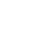学习PHP5个月了 总感觉自己学不进去 我应该报着什么样的心态去学习

年薪达不到25W大数据工程师、拿不到Offer全额退款->>>00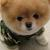0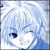0
0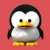<?php
function ve(\$var, \$i = '') {
\$indent = '  '.\$i;
if(is_array(\$var)) { //结束条件
echo "[\n";
foreach(\$var as \$k => \$v) {
echo \$indent;
if(is_array(\$v)) {
echo \$k." => ";
} else {
echo \$k;
}
if(\$k === 'GLOBALS') {
echo "*RECURSION*\n";
echo substr(\$indent, 0, -2)."]\n";
return;
} else {
ve(\$v, \$indent); //参数传递
}
}
echo substr(\$indent, 0, -2)."]\n";
} else {
if(\$indent == '  '){
echo \$var."\n"; //ve('php');
} else {
echo " => ".\$var."\n";
}
}
}
\$arr = array(
\$GLOBALS,
'abc',
'php' => 'best',
'win' => array(
'dis' => 1024,
'age' => array(
'a1' => 512,
'a2' => 15
)
),
'osx' => array(
'dis' => 512,
'age' => 15
),
'lin' => array(
'dis' => 512,
'age' => 25,
'lin' => array()
)
);
ve(\$arr); //输出
[
0 => [
_GET => [
]
_POST => [
]
]
_FILES => [
]
GLOBALS => *RECURSION*
]
1 => abc
php => best
win => [
dis => 1024
age => [
a1 => 512
a2 => 15
]
]
osx => [
dis => 512
age => 15
]
lin => [
dis => 512
age => 25
lin => [
]
]
]

RBAC(Role-Based Access Control,基于角色的访问控制):

user表每个用户都对应一个group.
group里每个字段就是一个权限,这样就可以开关不同的权限组合不同的用户组了.0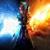0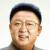0